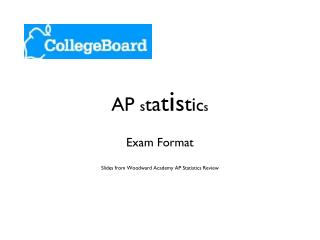DownloadDownload PresentationAP s t a t i s t i c s

AP s t a t i s t i c s

Télécharger la présentationAP s t a t i s t i c s

- - - - - - - - - - - - - - - - - - - - - - - - - - - E N D - - - - - - - - - - - - - - - - - - - - - - - - - - -
Presentation Transcript

1. AP statistics Exam Format Slides from Woodward Academy AP Statistics Review

2. I. Exploring Data Describing patterns and departures from patterns (20%-30%) Exploring analysis of data makes use of graphical and numerical techniques to study patterns and departures from patterns. Emphasis should be placed on interpreting information from graphical and numerical displays and summaries.

3. I. Exploring Data • Constructing and interpreting graphical displays of distributions of univariate data (dotplot, stemplot, histogram, cumulative frequency plot) • Center and spread • Clusters and gaps • Outliers and other unusual features • Shape

4. I. Exploring Data • Summarizing distributions of univariate data • Measuring center: median, mean • Measuring spread: range, interquartile range, standard deviation • Measuring position: quartiles, percentiles, standardized scores (z-scores) • Using boxplots • The effect of changing units on summary measures

5. I. Exploring Data • Comparing distributions of univariate data (dotplots, back-to-back stemplots, parallel boxplots) • Comparing center and spread: within group, between group variables • Comparing clusters and gaps • Comparing outliers and other unusual features • Comparing shapes

6. I. Exploring Data • Exploring bivariate data • Analyzing patterns in scatterplots • Correlation and linearity • Least-squares regression line • Residuals plots, outliers, and influential points • Transformations to achieve linearity: logarithmic and power transformations

7. I. Exploring Data • Exploring categorical data • Frequency tables and bar charts • Marginal and joint frequencies for two-way tables • Conditional relative frequencies and association • Comparing distributions using bar charts

8. II. Sampling and Experimentation Planning and conducting a study (10%-15%) Data must be collected according to a well-developed plan if valid information on a conjecture is to be obtained. This includes clarifying the question and deciding upon a method of data collection and analysis.

9. II. Sampling and Experimentation • Overview of methods of data collection • Census • Sample survey • Experiment • Observational study

10. II. Sampling and Experimentation • Planning and conducting surveys • Characteristics of a well-designed and well-conducted survey • Populations, samples, and random selection • Sources of bias in sampling and surveys • Sampling methods, including simple random sampling, stratified random sampling, and cluster sampling

11. II. Sampling and Experimentation • Planning and conducting experiments • Characteristics of a well-designed and well-conducted experiment • Treatments, control groups, experimental units, random assignments, and replication • Sources of bias and confounding, including placebo effect and blinding • Randomized block design, including matched pairs design

12. II. Sampling and Experimentation • Generalizability of results and types of conclusions that can be drawn from observational studies, experiments, and surveys

13. III. Anticipating Patterns Exploring random phenomena using probability and simulation (20%-30%) Probability is the tool used for anticipating what the distribution of data should look like under a given model.

14. III. Anticipating Patterns • Probability • Interpreting probability, including long-run relative frequency interpretation • “Law of Large Numbers” concept • Addition rule, multiplication rule, conditional probability, and independence • Discrete random variables and their probability distributions, including binomial and geometric • Simulation of random behavior and probability distributions • Mean (expected value) and standard deviation of a random variable and linear transformation of a random variable

15. III. Anticipating Patterns • Combining independent random variables • Notion of independence versus dependence • Mean and standard deviation for sums and differences of independent random variables

16. III. Anticipating Patterns • The normal distribution • Properties of the normal distribution • Using tables of the normal distribution • The normal distribution as a model for measurements

17. III. Anticipating Patterns • Sampling distributions • Sampling distribution of a sample proportion • Sampling distribution of a sample mean • Central Limit Theorem • Sampling distribution of a difference between two independent sample proportions • Sampling distribution of a difference between two independent sample means • Simulation of sampling distributions • t-distribution • Chi-square distribution

18. IV. Statistical Inference Estimating population parameters and testing hypotheses (30%-40%) Statistical inference guides the selection of appropriate models.

19. IV. Statistical Inference • Estimation (point estimators and confidence intervals) • Estimating population parameters and margins of error • Properties of point estimators, including unbiasedness and variability • Logic of confidence intervals, meaning of confidence level and intervals, and properties of confidence intervals • Large sample confidence interval for a proportion • Large sample confidence interval for the difference between two proportions • Confidence interval for a mean • Confidence interval for the difference between two means (unpaired and paired) • Confidence interval for the slope of a least-squares regression line

20. IV. Statistical Inference • Tests of Significance • Logic of significance testing, null and alternative hypotheses; p-values; one- and two-sided tests; concepts of Type I and Type II errors; concept of power • Large sample test for a proportion • Large sample test for a difference between two proportions • Test for a mean • Test for a difference between two means (unpaired and paired) • Chi-square test for goodness of fit, homogeneity of proportions, and independence (one- and two-way tables) • Test for the slope of a least-squares regression line